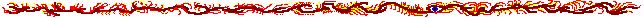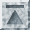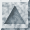# Data equalizer

This is a web-based data equalization software. Its purpose is to preprocess and postprocess data for learning algorithms such as neural networks. It is more generally applicable to other classifier systems, prediction methods, etc.

The software behind this service is based on the Inanna neural learning C++ library, available under the LGPL license (GNU Library General Public License) from http://inanna.sourceforge.net/.

### 1. Equalization parameters

Equalization method:
Gaussian

Linear range

Histogram
Parameters:
 Average = StdDev =
 Min = Max =
 Min = Max = Resolution =
Description: Linear method that moves the average of the original data to Average (default 0.0) in the equalized data, and scales the values so that values at the standard deviation will be scaled to -StdDev (default -1.0) and StdDev (default 1.0). Performs linear scaling so that the minimum of the analyzed data will be Min (default 0.0) and the maximum will be Max (default 1.0). Nonlinear method that equalizes the distribution of the data to uniform distribution in range from Min to Max (default 0.0-1.0). The analysis of the distribution is done with a histogram that has Resolution values (default 100000).

 Direction: Equalize Unequalize The data can either be equalized or unequalized. Unequalization is typically used for result data from a function estimation or prediction method, which you want to return to the original value range. Missing values: Leave as they are Set to average Missing values are indicated in data with 'x' values. This designation can be preserved during equalization, or they can be set to the average of the output of the equalization method. With gaussian method this will be Average, with linear range and histogram methods (Max-Min)/2. Analysis scale: Per column Global The analysis can be done separately with each column or globally with all the columns. The global equalization may be useful, if all the columns in the original data share same unit of measure (for example, dollars).

### 2. Data to be analyzed

This dataset will be analyzed and then equalized. It is typically training data for a learning system.

Format

• The data must be given in newline-separated rows.
• Empty or otherwise malformed rows are not allowed.
• The rows must have equal number of fields separated by one or more whitespace characters (space, ASCII 0x20; or horizonal tabulator, ASCII 0x09).
• Missing values are indicated with value 'x'.
• Fields may not be empty, but must contain a numeric value, or a missing-value indicator.
• Floating-point values must use dot '.' as their point separator. They may use exponential expression such as '10e-10'.
• Other characters are interpreted as beginning of comment.

Equalize also this analyzed data. If the analyzed data is used as training data for a learning method, you definitely want to equalize it too. Notice that unequalization will not, in any case, be applied to the analysis data.

### 3. Data to be equalized (optional)

This data will be equalized according to the analysis based on the above data. It is typically test data or validation data.

Format. Format is same as for the analysis data.

• Each row must have exactly the same number of values as in analysis data.
• Multiple datasets can be separated with an empty row.

### 4. Output type

Select output type:

text/html will be nicely formatted HTML output. text/plain is plain text displayed in browser, and with application/text you will be propted to save it on disk.

### 5. Submit request

The equalization request will be sent to the server application. This may take some time if the data is very large.

Report any problems to magi@iki.fi, because if you encounter any, I probably don't know about them, and they need to be fixed! Thank you!Last modified: Fri May 14 13:44:49 EEST 2004